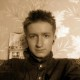Ask Your Question

# Make Border and sum

I want to create a border around an image, but only on the bottom and the right, with zeros with the purpose of doing a DFT. I have this piece of code:

cv::Mat computeDFT(cv::Mat image, int optimal_rows, int optimal_cols) {
cv::Mat padded;

std::cout << image.rows << " " << image.cols << " " << image.type() << " " << cv::sum(image) << std::endl;

cv::copyMakeBorder(image, padded, 0, optimal_rows - image.rows, 0, optimal_cols - image.cols, cv::BORDER_CONSTANT, cv::Scalar(0));

std::cout << padded.rows << " " << padded.cols << " " << padded.type() << " " << cv::sum(padded) << std::endl;

cv::Mat complexImage(padded.rows, padded.cols, CV_64FC2);
cv::dft(padded, complexImage, CV_HAL_DFT_COMPLEX_OUTPUT);
return complexImage;
}


The strange thing is that when i call this function with a motion kernel as image with cos and sines as data, the output is this:

65 65 6 [1, 0, 0, 0]
482 600 6 [0.99999999999999956, 0, 0, 0]


First one makes sense as it's a 65x65x1 kernel (6 = CV_64FC1), while in the second one i don't have the same sum. Anybody know why?

edit retag close merge delete

## 1 answer

Sort by » oldest newest most votedBecause in your particular case copyMakeBorder makes extrapolation, as a result you have got slightly different sum value. To prevent this you can make custom copy without extrapolation:

cv::Mat computeDFT(cv::Mat image, int optimal_rows, int optimal_cols) {
cv::Mat padded = cv::Mat(optimal_rows, optimal_cols, image.type());

std::cout << image.rows << " " << image.cols << " " << image.type() << " " << cv::sum(image) << std::endl;

cv::Mat _tempmat = cv::Mat(padded, cv::Rect(0,0,image.cols,image.rows));
image.copyTo(_tempmat);

std::cout << padded.rows << " " << padded.cols << " " << padded.type() << " " << cv::sum(padded) << std::endl;

cv::Mat complexImage(padded.rows, padded.cols, CV_64FC2);
cv::dft(padded, complexImage, CV_HAL_DFT_COMPLEX_OUTPUT);
return complexImage;
}

more

## Comments

After the copyTo call, which Mat has the new padded Array? Because the print after the call still shows the 0.999.. value

image Mat copies themself into the part of the padded Mat. If 0.999... still appears the result comes from the float point arithmetic features, try to check if it will still be 0.999... if you'll increase optimal_rows and optimal_cols by 1

Official site

GitHub

Wiki

Documentation

## Stats

Asked: 2017-02-22 03:41:13 -0500

Seen: 190 times

Last updated: Feb 22 '17